# sklearn.ensemble.HistGradientBoostingRegressor¶

class sklearn.ensemble.HistGradientBoostingRegressor(loss='squared_error', *, quantile=None, learning_rate=0.1, max_iter=100, max_leaf_nodes=31, max_depth=None, min_samples_leaf=20, l2_regularization=0.0, max_bins=255, categorical_features=None, monotonic_cst=None, interaction_cst=None, warm_start=False, early_stopping='auto', scoring='loss', validation_fraction=0.1, n_iter_no_change=10, tol=1e-07, verbose=0, random_state=None)[source]

This estimator is much faster than GradientBoostingRegressor for big datasets (n_samples >= 10 000).

This estimator has native support for missing values (NaNs). During training, the tree grower learns at each split point whether samples with missing values should go to the left or right child, based on the potential gain. When predicting, samples with missing values are assigned to the left or right child consequently. If no missing values were encountered for a given feature during training, then samples with missing values are mapped to whichever child has the most samples.

This implementation is inspired by LightGBM.

Read more in the User Guide.

New in version 0.21.

Parameters:
loss{‘squared_error’, ‘absolute_error’, ‘gamma’, ‘poisson’, ‘quantile’}, default=’squared_error’

The loss function to use in the boosting process. Note that the “squared error”, “gamma” and “poisson” losses actually implement “half least squares loss”, “half gamma deviance” and “half poisson deviance” to simplify the computation of the gradient. Furthermore, “gamma” and “poisson” losses internally use a log-link, “gamma” requires y > 0 and “poisson” requires y >= 0. “quantile” uses the pinball loss.

Changed in version 0.23: Added option ‘poisson’.

Changed in version 1.1: Added option ‘quantile’.

Changed in version 1.3: Added option ‘gamma’.

quantilefloat, default=None

If loss is “quantile”, this parameter specifies which quantile to be estimated and must be between 0 and 1.

learning_ratefloat, default=0.1

The learning rate, also known as shrinkage. This is used as a multiplicative factor for the leaves values. Use 1 for no shrinkage.

max_iterint, default=100

The maximum number of iterations of the boosting process, i.e. the maximum number of trees.

max_leaf_nodesint or None, default=31

The maximum number of leaves for each tree. Must be strictly greater than 1. If None, there is no maximum limit.

max_depthint or None, default=None

The maximum depth of each tree. The depth of a tree is the number of edges to go from the root to the deepest leaf. Depth isn’t constrained by default.

min_samples_leafint, default=20

The minimum number of samples per leaf. For small datasets with less than a few hundred samples, it is recommended to lower this value since only very shallow trees would be built.

l2_regularizationfloat, default=0

The L2 regularization parameter. Use 0 for no regularization (default).

max_binsint, default=255

The maximum number of bins to use for non-missing values. Before training, each feature of the input array X is binned into integer-valued bins, which allows for a much faster training stage. Features with a small number of unique values may use less than max_bins bins. In addition to the max_bins bins, one more bin is always reserved for missing values. Must be no larger than 255.

categorical_featuresarray-like of {bool, int, str} of shape (n_features) or shape (n_categorical_features,), default=None

Indicates the categorical features.

• None : no feature will be considered categorical.

• boolean array-like : boolean mask indicating categorical features.

• integer array-like : integer indices indicating categorical features.

• str array-like: names of categorical features (assuming the training data has feature names).

For each categorical feature, there must be at most max_bins unique categories, and each categorical value must be in [0, max_bins -1]. During prediction, categories encoded as a negative value are treated as missing values.

Read more in the User Guide.

New in version 0.24.

Changed in version 1.2: Added support for feature names.

monotonic_cstarray-like of int of shape (n_features) or dict, default=None

Monotonic constraint to enforce on each feature are specified using the following integer values:

• 1: monotonic increase

• 0: no constraint

• -1: monotonic decrease

If a dict with str keys, map feature to monotonic constraints by name. If an array, the features are mapped to constraints by position. See Using feature names to specify monotonic constraints for a usage example.

The constraints are only valid for binary classifications and hold over the probability of the positive class. Read more in the User Guide.

New in version 0.23.

Changed in version 1.2: Accept dict of constraints with feature names as keys.

interaction_cst{“pairwise”, “no_interaction”} or sequence of lists/tuples/sets of int, default=None

Specify interaction constraints, the sets of features which can interact with each other in child node splits.

Each item specifies the set of feature indices that are allowed to interact with each other. If there are more features than specified in these constraints, they are treated as if they were specified as an additional set.

The strings “pairwise” and “no_interactions” are shorthands for allowing only pairwise or no interactions, respectively.

For instance, with 5 features in total, interaction_cst=[{0, 1}] is equivalent to interaction_cst=[{0, 1}, {2, 3, 4}], and specifies that each branch of a tree will either only split on features 0 and 1 or only split on features 2, 3 and 4.

New in version 1.2.

warm_startbool, default=False

When set to True, reuse the solution of the previous call to fit and add more estimators to the ensemble. For results to be valid, the estimator should be re-trained on the same data only. See the Glossary.

early_stopping‘auto’ or bool, default=’auto’

If ‘auto’, early stopping is enabled if the sample size is larger than 10000. If True, early stopping is enabled, otherwise early stopping is disabled.

New in version 0.23.

scoringstr or callable or None, default=’loss’

Scoring parameter to use for early stopping. It can be a single string (see The scoring parameter: defining model evaluation rules) or a callable (see Defining your scoring strategy from metric functions). If None, the estimator’s default scorer is used. If scoring='loss', early stopping is checked w.r.t the loss value. Only used if early stopping is performed.

validation_fractionint or float or None, default=0.1

Proportion (or absolute size) of training data to set aside as validation data for early stopping. If None, early stopping is done on the training data. Only used if early stopping is performed.

n_iter_no_changeint, default=10

Used to determine when to “early stop”. The fitting process is stopped when none of the last n_iter_no_change scores are better than the n_iter_no_change - 1 -th-to-last one, up to some tolerance. Only used if early stopping is performed.

tolfloat, default=1e-7

The absolute tolerance to use when comparing scores during early stopping. The higher the tolerance, the more likely we are to early stop: higher tolerance means that it will be harder for subsequent iterations to be considered an improvement upon the reference score.

verboseint, default=0

The verbosity level. If not zero, print some information about the fitting process.

random_stateint, RandomState instance or None, default=None

Pseudo-random number generator to control the subsampling in the binning process, and the train/validation data split if early stopping is enabled. Pass an int for reproducible output across multiple function calls. See Glossary.

Attributes:
do_early_stopping_bool

Indicates whether early stopping is used during training.

n_iter_int

Number of iterations of the boosting process.

n_trees_per_iteration_int

The number of tree that are built at each iteration. For regressors, this is always 1.

train_score_ndarray, shape (n_iter_+1,)

The scores at each iteration on the training data. The first entry is the score of the ensemble before the first iteration. Scores are computed according to the scoring parameter. If scoring is not ‘loss’, scores are computed on a subset of at most 10 000 samples. Empty if no early stopping.

validation_score_ndarray, shape (n_iter_+1,)

The scores at each iteration on the held-out validation data. The first entry is the score of the ensemble before the first iteration. Scores are computed according to the scoring parameter. Empty if no early stopping or if validation_fraction is None.

is_categorical_ndarray, shape (n_features, ) or None

Boolean mask for the categorical features. None if there are no categorical features.

n_features_in_int

Number of features seen during fit.

New in version 0.24.

feature_names_in_ndarray of shape (n_features_in_,)

Names of features seen during fit. Defined only when X has feature names that are all strings.

New in version 1.0.

GradientBoostingRegressor

Exact gradient boosting method that does not scale as good on datasets with a large number of samples.

sklearn.tree.DecisionTreeRegressor

A decision tree regressor.

RandomForestRegressor

A meta-estimator that fits a number of decision tree regressors on various sub-samples of the dataset and uses averaging to improve the statistical performance and control over-fitting.

AdaBoostRegressor

A meta-estimator that begins by fitting a regressor on the original dataset and then fits additional copies of the regressor on the same dataset but where the weights of instances are adjusted according to the error of the current prediction. As such, subsequent regressors focus more on difficult cases.

Examples

>>> from sklearn.ensemble import HistGradientBoostingRegressor
>>> est.score(X, y)
0.92...


Methods

 fit(X, y[, sample_weight]) Fit the gradient boosting model. get_params([deep]) Get parameters for this estimator. Predict values for X. score(X, y[, sample_weight]) Return the coefficient of determination of the prediction. set_params(**params) Set the parameters of this estimator. Predict regression target for each iteration.
fit(X, y, sample_weight=None)[source]

Parameters:
Xarray-like of shape (n_samples, n_features)

The input samples.

yarray-like of shape (n_samples,)

Target values.

sample_weightarray-like of shape (n_samples,) default=None

Weights of training data.

New in version 0.23.

Returns:
selfobject

Fitted estimator.

get_params(deep=True)[source]

Get parameters for this estimator.

Parameters:
deepbool, default=True

If True, will return the parameters for this estimator and contained subobjects that are estimators.

Returns:
paramsdict

Parameter names mapped to their values.

property n_iter_

Number of iterations of the boosting process.

predict(X)[source]

Predict values for X.

Parameters:
Xarray-like, shape (n_samples, n_features)

The input samples.

Returns:
yndarray, shape (n_samples,)

The predicted values.

score(X, y, sample_weight=None)[source]

Return the coefficient of determination of the prediction.

The coefficient of determination $$R^2$$ is defined as $$(1 - \frac{u}{v})$$, where $$u$$ is the residual sum of squares ((y_true - y_pred)** 2).sum() and $$v$$ is the total sum of squares ((y_true - y_true.mean()) ** 2).sum(). The best possible score is 1.0 and it can be negative (because the model can be arbitrarily worse). A constant model that always predicts the expected value of y, disregarding the input features, would get a $$R^2$$ score of 0.0.

Parameters:
Xarray-like of shape (n_samples, n_features)

Test samples. For some estimators this may be a precomputed kernel matrix or a list of generic objects instead with shape (n_samples, n_samples_fitted), where n_samples_fitted is the number of samples used in the fitting for the estimator.

yarray-like of shape (n_samples,) or (n_samples, n_outputs)

True values for X.

sample_weightarray-like of shape (n_samples,), default=None

Sample weights.

Returns:
scorefloat

$$R^2$$ of self.predict(X) w.r.t. y.

Notes

The $$R^2$$ score used when calling score on a regressor uses multioutput='uniform_average' from version 0.23 to keep consistent with default value of r2_score. This influences the score method of all the multioutput regressors (except for MultiOutputRegressor).

set_params(**params)[source]

Set the parameters of this estimator.

The method works on simple estimators as well as on nested objects (such as Pipeline). The latter have parameters of the form <component>__<parameter> so that it’s possible to update each component of a nested object.

Parameters:
**paramsdict

Estimator parameters.

Returns:
selfestimator instance

Estimator instance.

staged_predict(X)[source]

Predict regression target for each iteration.

This method allows monitoring (i.e. determine error on testing set) after each stage.

New in version 0.24.

Parameters:
Xarray-like of shape (n_samples, n_features)

The input samples.

Yields:
ygenerator of ndarray of shape (n_samples,)

The predicted values of the input samples, for each iteration.

## Examples using sklearn.ensemble.HistGradientBoostingRegressor¶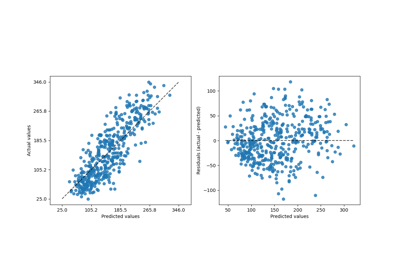Release Highlights for scikit-learn 1.2

Release Highlights for scikit-learn 1.2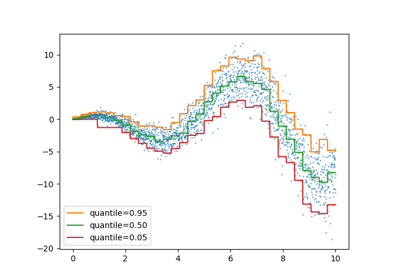Release Highlights for scikit-learn 1.1

Release Highlights for scikit-learn 1.1Release Highlights for scikit-learn 1.0

Release Highlights for scikit-learn 1.0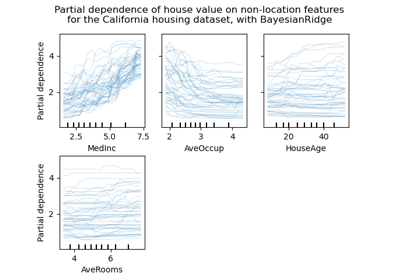Release Highlights for scikit-learn 0.24

Release Highlights for scikit-learn 0.24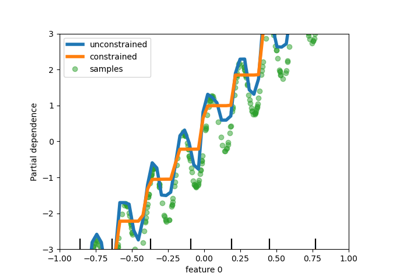Release Highlights for scikit-learn 0.23

Release Highlights for scikit-learn 0.23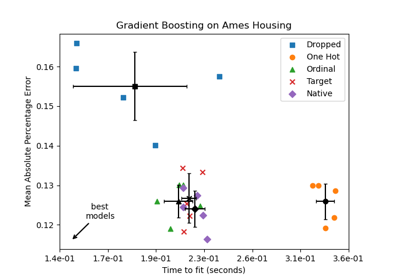Categorical Feature Support in Gradient Boosting

Categorical Feature Support in Gradient Boosting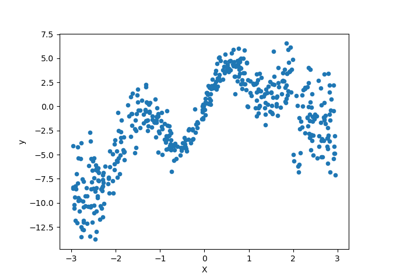Combine predictors using stacking

Combine predictors using stacking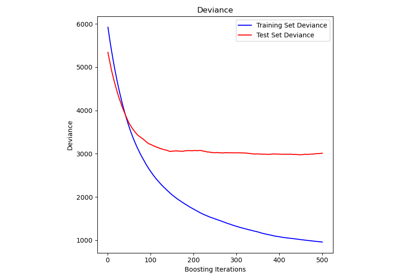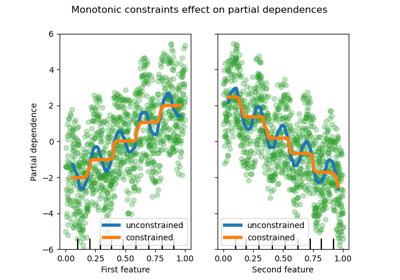Monotonic Constraints

Monotonic Constraints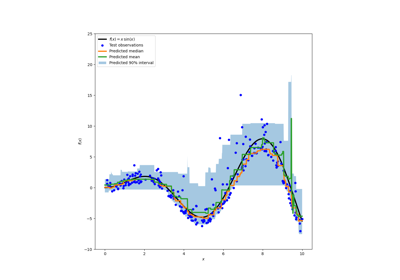Prediction Intervals for Gradient Boosting Regression

Prediction Intervals for Gradient Boosting Regression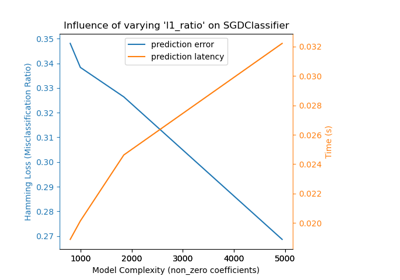Model Complexity Influence

Model Complexity Influence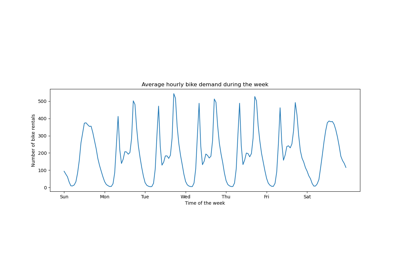Time-related feature engineering

Time-related feature engineering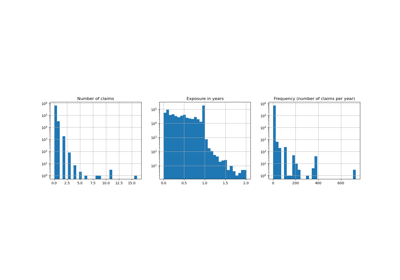Poisson regression and non-normal loss

Poisson regression and non-normal loss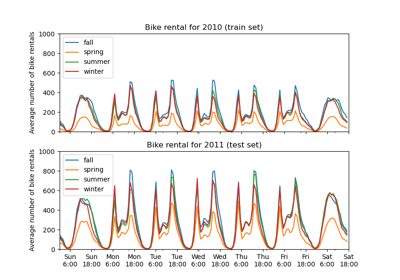Partial Dependence and Individual Conditional Expectation Plots

Partial Dependence and Individual Conditional Expectation Plots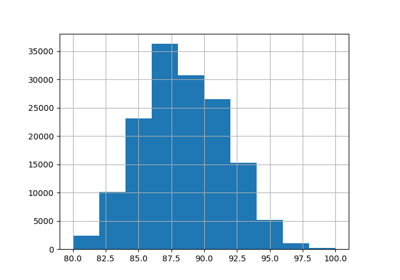Comparing Target Encoder with Other Encoders

Comparing Target Encoder with Other Encoders